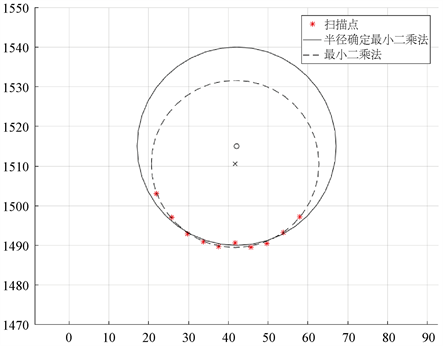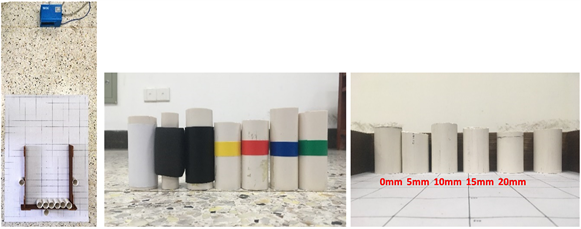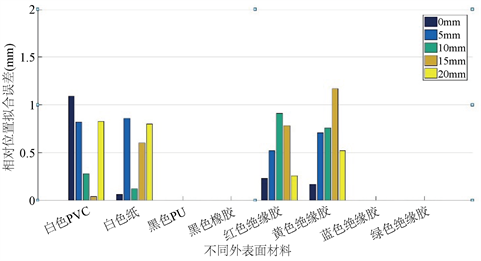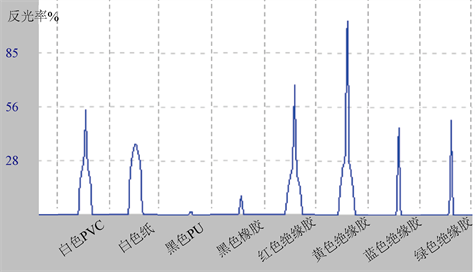﻿ 半径确定的最小二乘圆拟合法在电缆卷绕监测中的应用

# 半径确定的最小二乘圆拟合法在电缆卷绕监测中的应用Application of Radius-Determined Least Squares Circle Fitting Method in Cable Winding Monitoring

Abstract: Aiming at the problem of using only the least square method in the monitoring of the cable winding state and using insufficient cable specification information, an improved circle fitting method is designed: the least squares fitting method for radius determination. The effects of sensor angular resolution, cable diameter, cable outer surface material and other factors on the fitting accuracy of cable relative position were studied experimentally. The experimental results show that the main factor affecting the fitting accuracy is the accuracy of the measured data. For the cable with common black rubber appearance, when the random measurement error of the sensor is less than 7 mm, the algorithm can ensure that the fitting error of the relative position of the cable is less than 1.5 mm.

1. 引言

2. 数学原理

2.1. 一般的最小二乘圆拟合法

${\left(x-{x}_{0}\right)}^{2}+{\left(y-{y}_{0}\right)}^{2}={r}_{0}^{2}$ (1)

$F\left({x}_{0},{y}_{0},{r}_{0}\right)=\underset{i=1}{\overset{N}{\sum }}{\left[{\left({x}_{i}-{x}_{0}\right)}^{2}+{\left({y}_{i}-{y}_{0}\right)}^{2}-{r}_{0}^{2}\right]}^{2}$ (2)

2.2. 半径确定的最小二乘圆拟合法

$G\left({x}_{0},{y}_{0}\right)=\underset{i=1}{\overset{N}{\sum }}{\left[{\left({x}_{i}-{x}_{0}\right)}^{2}+{\left({y}_{i}-{y}_{0}\right)}^{2}-{R}_{0}^{2}\right]}^{2}$ (3)Figure 1. Comparison of fitting effects

3. 实验验证与分析

3.1. 角度分辨率对拟合精度的影响(a) (b)(c)

Figure 2. Cable winding simulation diagram. (a) The experiment site; (b) Tubes with different surface materials; (c) Different distances for testingTable 1. Relationship between sensor random error and reflectivityTable 2. Relative position fitting errors at different angular resolutionsFigure 3. Fitting error of different angular resolutions

3.2. 电缆直径对拟合精度的影响Figure 4. Fitting error of different cable diametersTable 3. Relative position fitting errors for different cable diameters

3.3. 外表面材料对拟合精度的影响Table 4. Relative position fitting errors of different outer surface materialsFigure 5. Fitting error of different outer surface materialsFigure 6. Reflectivity of different outer surface materialsTable 5. Comparison of fitting precision between white PVC and black rubber

4. 总结

 王江平, 田亮, 付忠文, 李钦彬. 井下设备电缆卷缆装置的改装设计[J]. 煤矿机械, 2011(9): 20-21.

 Arai, M. and Hirose, S. (2007) Improved Reel Mechanism to Wind a Cable Uniformly in Spherical Trailer. 2007 IEEE International Workshop on Safety, Security and Rescue Robotics, Rome, 27-29 September 2007, 1-6.
https://doi.org/10.1109/SSRR.2007.4381257

 陆海坤, 贾彦辉, 李聪聪. 新型差动电缆卷绕装置研究[J]. 河北省科学院学报, 2012(2): 37-40.

 杨洋, 李柯, 杨学敬, 俞友爱, 江涛, 顾菁宇. 电缆卷绕装置以及车载移动式检测设备[P]. 中国专利, 201710170091.7. 2017.03.21.

 程超, 范巍, 张静怡. 关于电缆卷绕过程的状态监测研究[J]. 传感器技术与应用, 2019, 7(3): 104-113.

 Shakarji, C.M. (1998) Least-Squares Fitting Algorithms of the NIST Algorithm Testing System. Journal of Research of the National Institute of Standards and Technology, 103, 633-641.
https://doi.org/10.6028/jres.103.043

 杜乐普, 金印, 石宏, 李伟楠. 半径确定的最小二乘圆拟合法在涡轮机匣检测中的应用[J]. 沈阳航空航天大学学报, 2014, 31(2): 24-27.

 刘珂, 周富强, 张广军. 半径约束最小二乘圆拟合方法及其误差分析[J]. 光电子•激光, 2006, 17(5): 604-607.

 朱嘉, 李醒飞, 谭文斌, 等. 基于圆心约束最小二乘圆拟合的短弧测量[J]. 光学精密工程, 2009, 17(10): 2487-2489.

Top